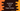# JavaScript program to find if a number is a Magic number or not## JavaScript program to find if a number is a Magic number or not:

In this post, we will learn how to find if a number is a Magic number or not with JavaScript. We will write a program that will take one number as input from the user, check if it is a Magic number and print out the result.

### What is a Magic number:

A number is called a Magic number if the repeated sum of digits of that number is equal to 1. 9721 is a Magic number. Let me show you how:

• The sum of digits of 9721 is 9 + 7 + 2 + 1 or 19.
• Since 19 is a two digit number, we have to find the sum of digits of 19 which is 10. Similarly, the sum of digits of 10 is 1. Hence, the repeated sum of digits of the number is equal to 1 and so 9721 is a Magic number.

Similarly, 2179, 10 etc. are Magic numbers.

We will have to find the repeated sum of digits of a number first and if we compare it with 1, we can find if a number is a Magic number or not.

### Example 1: Magic number program with a while loop:

The following program uses a while loop to check if a number is Magic number or not:

``````let no = 9721;
let copyNo = no;
let sum = no;

while(copyNo > 9){
sum = 0;
while(copyNo > 0){
sum += copyNo%10;
copyNo = parseInt(copyNo/10);
}
copyNo = sum;
}

if(sum == 1){
console.log(`\${no} is a Magic number`)
}else{
console.log(`\${no} is not a Magic number`)
}``````

In this program,

• The variable no holds the given number. We created a copy of the number, copyNo. The program works on this copied number. It also initialized another sum variable to hold the repeated sum.
• There are two while loops used in the program.
• The outer loop runs until the value of copyNo is greater than 9. Inside this loop, it finds the sum of digits of the number and assigns that sum to copyNo. So, at the end of this loop, the sum variable will hold the repeated sum of digits of the number.
• The inner loop finds the sum of digits of the number copyNo. It finds the last digit of the number, adds it to the sum variable and removes it from copyNo.
• Once the loop ends, we can compare the value of the sum variable with 1. If it is equal to 1, it is a Magic number. Else, it is not a Magic number.

You can try this program with different numbers to check for Magic numbers.

### Example 2: Magic number program with HTML, JS:

We can also write this program with HTML and JavaScript. Create one index.html file with the below content:

``````<!DOCTYPE html>
<html lang="en">

<script>
function checkMagic() {
let no = document.getElementById("no").value;
let copyNo = no;
let sum = no;

while (copyNo > 9) {
sum = 0;
while (copyNo > 0) {
sum += copyNo % 10;
copyNo = parseInt(copyNo / 10);
}
copyNo = sum;
}
if (sum == 1) {
document.getElementById("result").innerText = `\${no} is a Magic number`;
} else {
document.getElementById("result").innerText = `\${no} is not a Magic number`;
}
}
</script>

<body>
<input type="text" id="no" placeholder="Enter the number" />
<button onclick="checkMagic()">Check</button>
<text id="result"></text>
</body>

</html>``````

You can open this file in any web browser to check the result.

• It has one input field to take the number input. The user will enter the number in that field.
• One button is there. On clicking the button, it will call the checkMagic function.
• The checkMagic function checks if the entered number is a Magic number or not.
• It updates the result in the text field.

It will give result as below:

### Example 3: Find all Magic numbers in a range in JavaScript:

Let’s change the above program to find all Magic numbers in a range with JavaScript.

``````function isMagic(no) {
let copyNo = no;
let sum = no;

while (copyNo > 9) {
sum = 0;
while (copyNo > 0) {
sum += copyNo % 10;
copyNo = parseInt(copyNo / 10);
}
copyNo = sum;
}
return sum == 1;
}

console.log(`Magic numbers between 1 and 100 are: `);
for(let i = 1; i <= 100; i++){
if(isMagic(i)){
console.log(i);
}
}``````

We moved the code to a new function isMagic. This function takes one number as its parameter and returns one boolean value. It is finding all magic numbers in between 1 and 100 by using a for loop. The loop runs from 1 to 100 and on each iteration, it checks if the current value is a Magic number or not. If yes, it prints that value.

It prints the below output:

``````Magic numbers between 1 and 100 are:
1
10
19
28
37
46
55
64
73
82
91
100``````